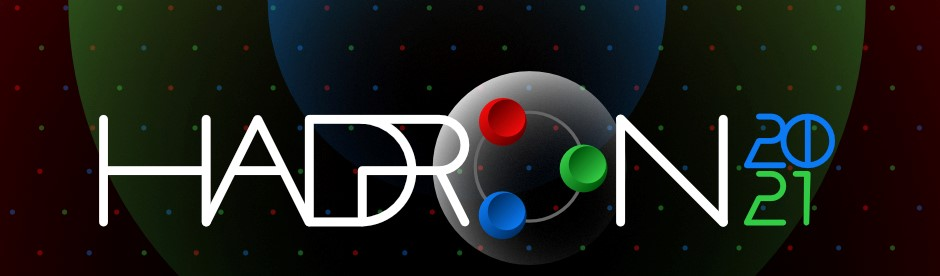#19th International Conference on Hadron Spectroscopy and Structure in memoriam Simon Eidelman

26-31 July 2021
Mexico City
Mexico/General timezone
HADRON 2021 is over. Thanks for making it a success!
Home > Timetable > Session details > Contribution details

# Contribution Parallel

Mexico City -
Meson Spectroscopy

# The $D {\bar{D}}$ bound state in the $\psi (3770) \rightarrow \gamma D^0 {\bar{D}}^0$ decay

## Speakers

• Dr. Genaro TOLEDO

## Abstract

We compute the $\Psi/3770) \to \gamma D \bar D$ decay, for both the $D^+ D^-$ and $D^0 \bar D^0$ decays. The $\Psi(3770) \to \gamma D^0 \bar D^0$ transition to go through a loop involving the $D^+ D^- \to D^0 \bar D^0$ scattering amplitude. We take the results for this amplitude from a theoretical model that predicts a $D \bar D$ bound state and find a $D^0 \bar D^0$ mass distribution in the decay drastically different than phase space.